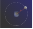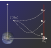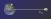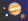Gravitational Physics

In light of the results of the last quiz, we know that the force of gravity is proportional to the product of the masses of both objects, $F_g \propto m_1 m_2.$

Newton expected this mass-dependence was only one part of a universal law of gravity. The distance of separation $r$ between two objects should also affect the gravitational force. This distance-dependence can be wrapped in an unknown function $f(r),$ and we can write the gravitational force between two masses $m_1$ and $m_2$ as $F_g=G m_1 m_2 f(r).$ Our goal is to use data from observations to constrain this function. In this quiz we will follow a line of Newton's own reasoning to take a stab at what $f(r)$ could be.

In the Newton's model of $F_g$ you may recognize $G$ as Newton's constant, but for now we will interpret it as a constant of proportionality that depends on our system of units. In the next quiz, we will estimate $G$ from data collection from Jupiter's moons, and in the final quiz of this chapter, we will endow it with a physical meaning.

Explore: Distance Dependence

Newton's great innovation was that the Earth's gravitational pull was responsible for the motion of the moon. Under this assumption and armed with some basic observations of the moon's orbital motion, we can start to unravel $f(r),$ the distance-dependence in Newtonian gravity.Assuming the moon's orbit is circular with Earth at its center, estimate its acceleration, $a_\text{Moon},$ in $\si[per-mode=symbol]{\meter\per\second\squared}$.

Hint

It will help to recall that the acceleration of an object moving at a constant speed on a circle is $a=\frac{v^2}{R}.$ The distance between Earth's center and the Moon's center is roughly $R=\SI{3.8e5}{\kilo\meter}$ and the period of the Moon's orbit is about $27.3$ days.

Explore: Distance Dependence

The figure below depicts three examples of how $f(r)$ might vary as a function of the distance between Earth and Moon.In light of the last calculation of the Moon's acceleration, only one of the three options is viable. Which one?

Explore: Distance Dependence

Newton was unable to solve for $f(r)$ directly from $F_g=GM_Emf(r)$ because in Newton's time, Earth's mass $M_E$ was unknown.

However, because Newton was quite clever, he could calculate the ratio $Z=\frac{f(R_E)}{f(R_M)}.$Can you? What is the numerical value of $Z$?

Details

• Use $g=\SI[per-mode=symbol]{9.8}{\meter\per\second\squared}$ and the moon's orbital acceleration.

Explore: Distance Dependence

Scientific model building usually relies on fitting observations of an unknown function to one of several common functional forms. One such common form is a power law: $f(r)=r^p,$ where $p\neq 0.$

By assuming $Z=\frac{f(R_E)}{f(R_M)} = \frac{R_E^p}{R_M^p}$ find a prediction for the unknown power $p.$

Details

• Use $R_E=\SI{6.4e3}{\kilo\meter}$ and $R_M=\SI{3.8e5}{\kilo\meter}.$ Both were known fairly accurately in Newton's time.
• Round your answer to the nearest integer.

Explore: Distance Dependence

Newton devoted much of his life to deriving the effects of a power-law force $F_g\propto \frac{1}{r^2},$ which we now call an inverse-square law. By the time he had performed the previous calculation, he had already proved the observed orbits of planets can be derived from it. (We will do these proofs explicitly in a later chapter.)

Make no mistake: the calculation in this quiz in no way constitutes a rigorous justification of Newton's inverse-square law of gravity. Newton's theory is validated only if the inverse-square dependence can be deduced from any orbit; we have only checked that $F_g\propto 1/r^2$ for the Moon's orbit. In the next quiz we'll see if orbital data from Jupiter's moons also validate this conclusion.×